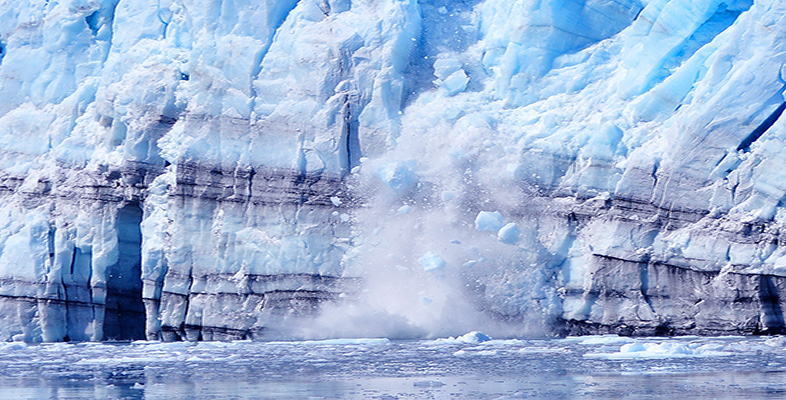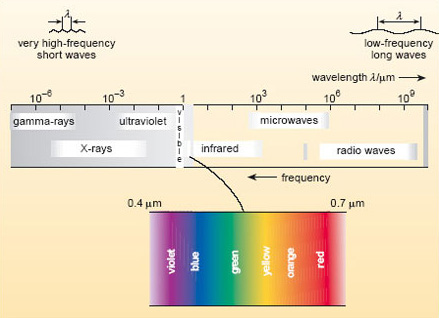Climate change

Start this free course now. Just create an account and sign in. Enrol and complete the course for a free statement of participation or digital badge if available.

# 1 Global climate and the greenhouse effect

## 1.1 Introduction

At the beginning of the 21st century, terms such as the 'greenhouse effect', 'greenhouse gases' and 'greenhouse warming' are printed or spoken thousands of times a week in the context of climate change caused by human activities. This section is designed to consolidate your understanding of the basic science behind these terms, and then to review what is known about the human impact on the composition of the atmosphere since the dawn of the industrial age, commonly put (in this context) at around AD 1750.

We start with a couple of fundamental questions about global climate. What determines the Earth's global mean surface temperature (GMST)? And how does the composition of the atmosphere come into that equation?

Electromagnetic radiation is the only form of energy transfer that travels through the vacuum of space, propagating as a wave. By convention, the full spectrum of electromagnetic radiation is carved up into regions, each characterised by a particular range of wavelengths (Figure 1). The wavelength (symbol λ ) is just the distance between successive crests of a wave.Figure 1 A portion of the electromagnetic spectrum. Wavelength is given in micrometres, μm: 1 μm = 10−6 m. Note that the wavelength changes by a factor of 10 for each division along the top scale, so this is a logarithmic scale.

Our eyes are sensitive to visible radiation, which corresponds to the wavelength range from about 0.4 μm (violet light) to 0.7 μm (red light). When all wavelengths in this range are present, we perceive this as 'white light'. To either side of the visible band lie the ranges known as ultraviolet (uv) radiation (with wavelengths below that of violet light) and infrared (ir) radiation (with wavelengths above that of red light).

As with any propagating waves, the shorter the wavelength, the higher the frequency (f ) (i.e. the higher the number of waves passing a point in a given time). For electromagnetic radiation, the two multiplied together give the speed of light (c): c =fλ .